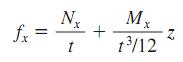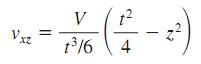Awarded as the best online publication by CIDC

# Building

Stresses In Thin Shells

Results of membrane and bending theories are expressed in terms of unit forces and unit moments, acting per unit of length over the thickness of the shell. To compute the unit stresses from these forces and moments, usual practice is to assume normal forces and shears to be uniformly distributed over the shell thickness and bending stresses to be linearly distributed. Then, normal stresses can be computed from equations of the form:where
z=distance from middle surface
t=shell thickness

Mx= unit bending moment about an axis parallel to direction of unit normal force Nx

Similarly, shearing stresses produced by central shears T and twisting moments D may be calculated from equations of the form:Normal shearing stresses may be computed on the assumption of a parabolic stress distribution over the shell thickness:where V=unit shear force normal to middle surface.

Allowable Stress Design For Shear in Buildings

The AISC specification for ASD specifies the following allowable shear stresses Fv, ksi ( ksiX6.894=MPa)

Load And Resistance Factor Design For Building Beams

For a compact section bent about the major axis, the unbraced length Lb of the compression flange, where plastic hinges may form at failure, may not exceed Lpd, given by Eqs. given in post. For beams bent about the minor axis and square and circular beams, Lb is not restricted for plastic analysis.

Allowable Stress Design For Building Beams

The maximum fiber stress in bending for laterally supported beams and girders is Fb = 0.66Fy if they are compact, except for hybrid girders and members with yield points exceeding 65 ksi (448.1 MPa). Fb = 0.60Fy for non-compact sections. Fy is the minimum specified yield strength of the steel, ksi (MPa). Table lists values of Fb for two grades of steel.

Load And Resistance Factor Design For Building Column

Plastic analysis of prismatic compression members in buildings is permitted if (Fy)½(l/r) does not exceed 800 and Fuless than equal to 65 ksi (448 MPa). For axially loaded members with b/t less than equal to (lambda)r , the maximum load P u , ksi (MPa= 6.894 X ksi), may be computed from

Pu = 0.85AgFcr

Where Ag=gross cross-sectional area of the member
F cr =0.658 lambda Fy for lambda <= 2.25

=0.877 Fy/lambda for lambda  > 2.25

lambda =(Kl/r)2(Fy/286,220)

The AISC specification for LRFD presents formulas for designing members with slender elements.